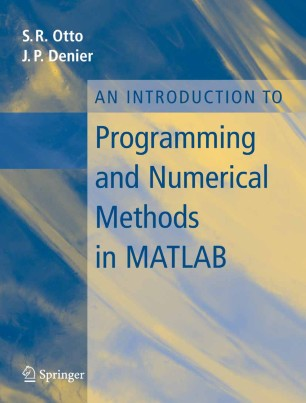# An Introduction to Programming and Numerical Methods in MATLAB

• S.R. Otto
• J.P. DenierTextbook

1. Front Matter
Pages i-xv
2. Pages 1-26
3. Pages 27-61
4. Pages 63-101
5. Pages 103-132
6. Pages 133-167
7. Pages 169-223
8. Pages 225-245
9. Pages 247-290
10. Pages 291-322
11. Back Matter
Pages 323-463

### Introduction

MATLAB is a powerful programme, which naturally lends itself to the rapid implementation of most numerical algorithms. This text, which uses MATLAB, gives a detailed overview of structured programming and numerical methods for the undergraduate student.

The book covers numerical methods for solving a wide range of problems, from integration to the numerical solution of differential equations or the stimulation of random processes. Examples of programmes that solve problems directly, as well as those that use MATLAB’s high-level commands are given.

Each chapter includes extensive examples and tasks, at varying levels of complexity. For practice, the early chapters include programmes that require debugging by the reader, while full solutions are given for all the tasks. The book also includes:

a glossary of MATLAB commands

appendices of mathematical techniques used in numerical methods.

Designed as a text for a first course in programming and algorithm design, as well as in numerical methods courses, the book will be of benefit to a wide range of students from mathematics and engineering, to commerce.

### Keywords

Interpolation MATLAB Numerical integration algorithms numerical methods programming

#### Authors and affiliations

• S.R. Otto
• 1
• J.P. Denier
• 2
1. 1.The R & ASt Andrews FifeScotland
2. 2.School of Mathematical SciencesThe University of AdelaideSouth AustraliaAustralia